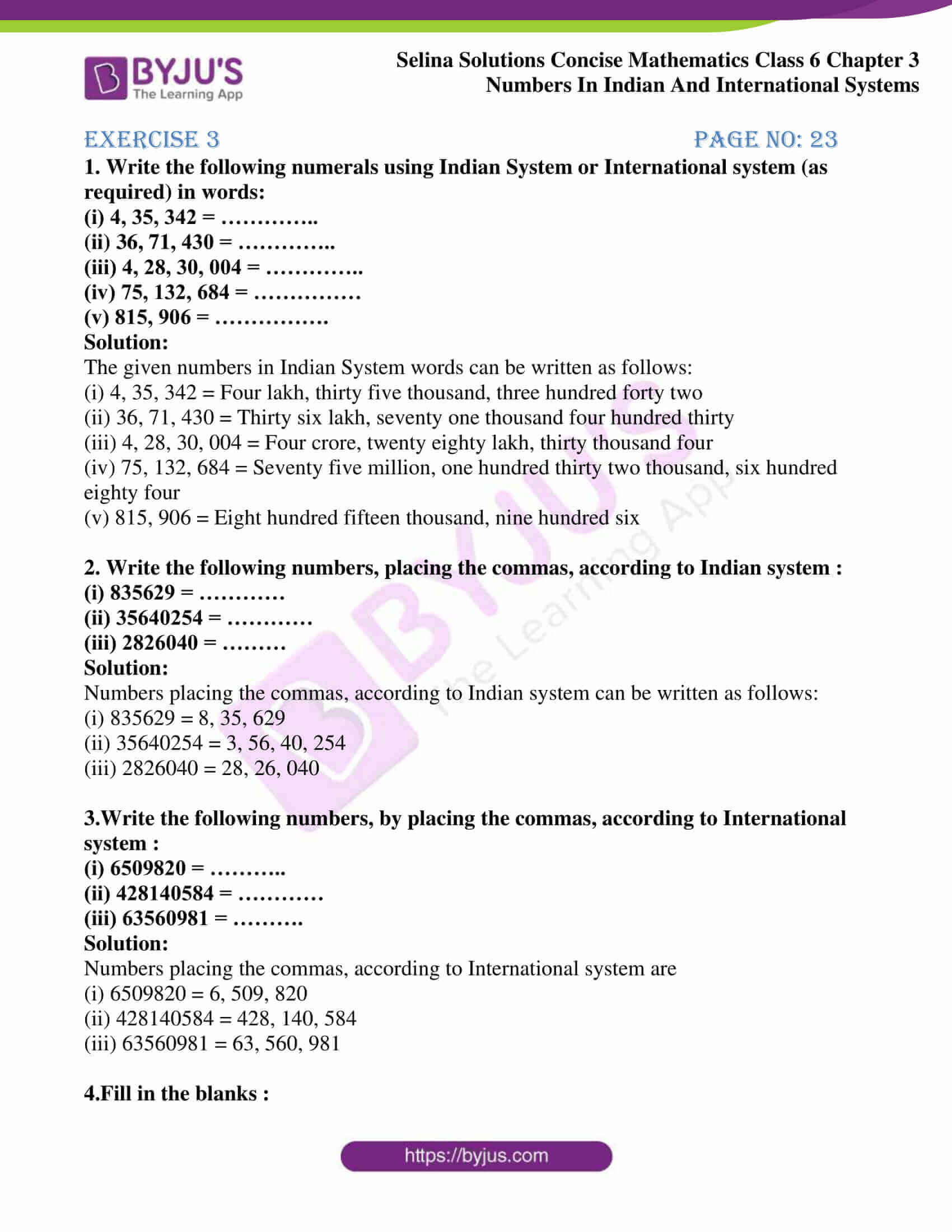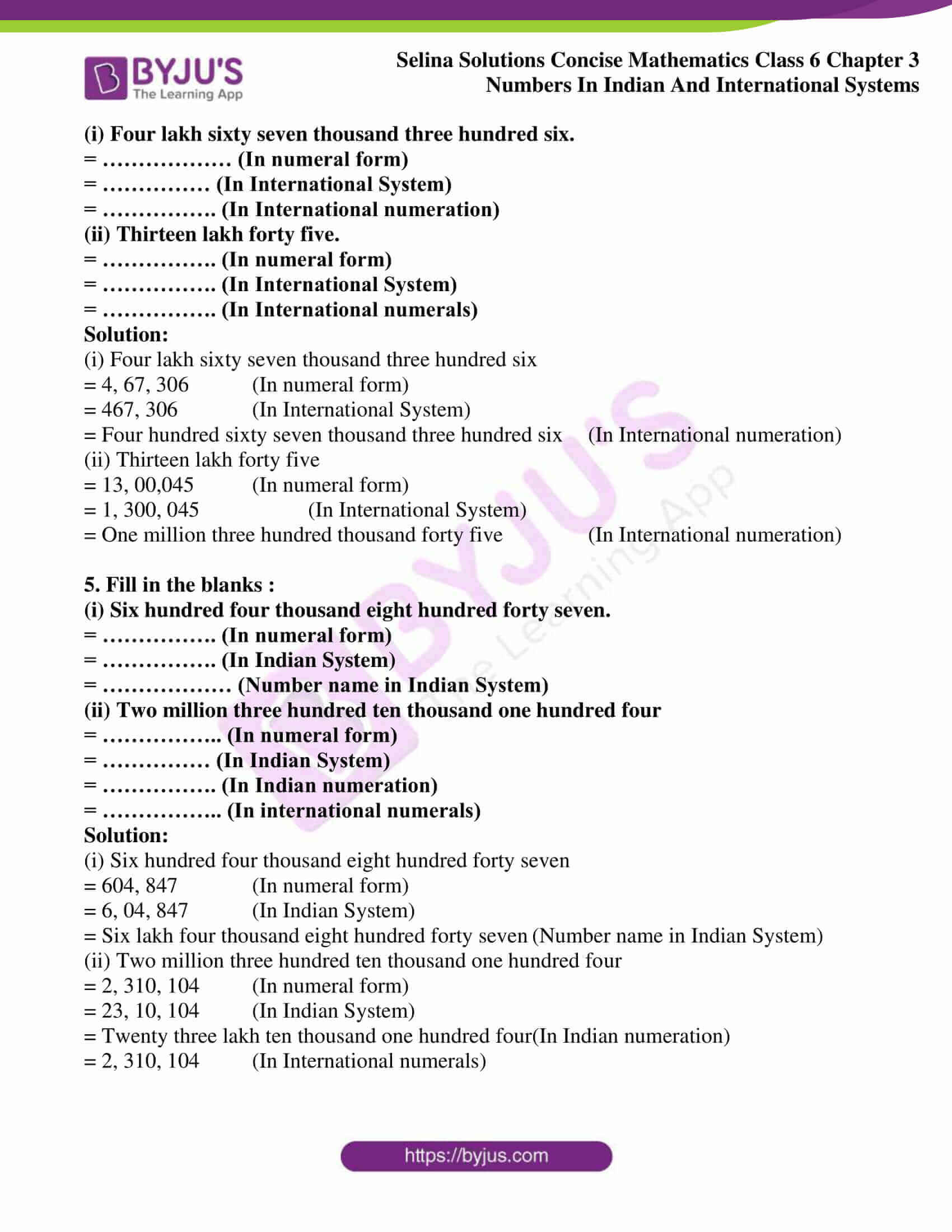# Selina Solutions Concise Mathematics Class 6 Chapter 3 Numbers In Indian And International Systems

Selina Solutions are the best study material among students as these Solutions are well structured with neat explanations. Students who practise these Solutions not only boost their confidence but also improve their academic performance in board exams. Experts provide the Solutions in such a way that students grasp them effortlessly and understand in a more effective way. Students become eager to learn Mathematics as they practise these Selina Solutions to boost exam preparation.

This chapter is based on unit, number, numeral and numeration. A symbol representing a given number is known as numeral and representing that number in words is known as numeration. Practising Selina Solutions helps students to obtain the method of solving complex problems with ease. Download the PDF of Selina Solutions Concise Mathematics Class 6 from the links provided here.

## Selina Solutions Concise Mathematics Class 6 Chapter 3: Numbers In Indian And International System Download PDF### Access Selina Solutions Concise Mathematics Class 6 Chapter 3: Numbers In Indian And International Systems

Exercise 3 page no: 23

1. Write the following numerals using Indian System or International system (as required) in words:
(i) 4, 35, 342 = …………..
(ii) 36, 71, 430 = …………..
(iii) 4, 28, 30, 004 = …………..
(iv) 75, 132, 684 = ……………
(v) 815, 906 = …………….

Solution:

The given numbers in Indian System words can be written as follows:

(i) 4, 35, 342 = Four lakh, thirty five thousand, three hundred forty two

(ii) 36, 71, 430 = Thirty six lakh, seventy one thousand four hundred thirty

(iii) 4, 28, 30, 004 = Four crore, twenty eighty lakh, thirty thousand four

(iv) 75, 132, 684 = Seventy five million, one hundred thirty two thousand, six hundred eighty four

(v) 815, 906 = Eight hundred fifteen thousand, nine hundred six

2. Write the following numbers, placing the commas, according to Indian system :
(i) 835629 = …………
(ii) 35640254 = …………
(iii) 2826040 = ………

Solution:

Numbers placing the commas, according to Indian system can be written as follows:

(i) 835629 = 8, 35, 629

(ii) 35640254 = 3, 56, 40, 254

(iii) 2826040 = 28, 26, 040

3.Write the following numbers, by placing the commas, according to International system :
(i) 6509820 = ………..
(ii) 428140584 = …………
(iii) 63560981 = ……….

Solution:

Numbers placing the commas, according to International system are

(i) 6509820 = 6, 509, 820

(ii) 428140584 = 428, 140, 584

(iii) 63560981 = 63, 560, 981

4.Fill in the blanks :
(i) Four lakh sixty seven thousand three hundred six.
= ……………… (In numeral form)
= …………… (In International System)
= ……………. (In International numeration)
(ii) Thirteen lakh forty five.
= ……………. (In numeral form)
= ……………. (In International System)
= ……………. (In International numerals)
Solution:

(i) Four lakh sixty seven thousand three hundred six

= 4, 67, 306 (In numeral form)

= 467, 306 (In International System)

= Four hundred sixty seven thousand three hundred six (In International numeration)

(ii) Thirteen lakh forty five

= 13, 00,045 (In numeral form)

= 1, 300, 045 (In International System)

= One million three hundred thousand forty five (In International numeration)

5. Fill in the blanks :
(i) Six hundred four thousand eight hundred forty seven.
= ……………. (In numeral form)
= ……………. (In Indian System)
= ……………… (Number name in Indian System)
(ii) Two million three hundred ten thousand one hundred four
= …………….. (In numeral form)
= …………… (In Indian System)
= ……………. (In Indian numeration)
= …………….. (In international numerals)

Solution:

(i) Six hundred four thousand eight hundred forty seven

= 604, 847 (In numeral form)

= 6, 04, 847 (In Indian System)

= Six lakh four thousand eight hundred forty seven (Number name in Indian System)

(ii) Two million three hundred ten thousand one hundred four

= 2, 310, 104 (In numeral form)

= 23, 10, 104 (In Indian System)

= Twenty three lakh ten thousand one hundred four (In Indian numeration)

= 2, 310, 104 (In International numerals)